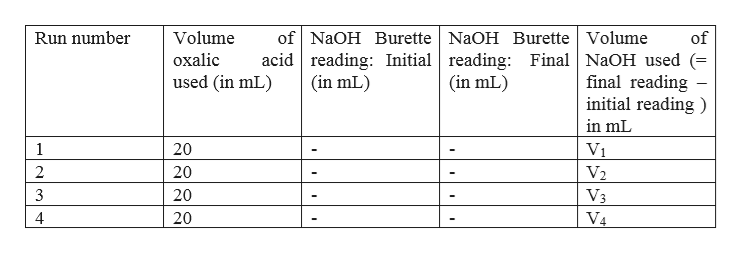In the lab, each group will be given a NaOH solution and a vial of solid H2C2O4·2H2O. Design a procedure to determine the molarity of the NaOH solution.

Question

In the lab, each group will be given a NaOH solution and a vial of solid H2C2O4·2H2O. Design a procedure to determine the molarity of the NaOH solution.

Step 1

As in the question it has not been mentioned anything about the strength of NaOH solution and oxalic acid, suppose the strength of given NaOH solution is 0.1 N. The first step is to prepare suppose 0.1 N oxalic acid from the given amount of solid oxalic acid.

Oxalic acid is a dibasic acid and generally available in crystalline form with 2 moles of water. Its molar mass is 126 g/mol. Its equivalent weight = (molecular weight / 2) = 63 g.

So to prepare 1000 mL of 1 N oxalic acid solution, the amount of oxalic acid required is 63 g. To prepare 1000 mL 0.1 N oxalic acid solution, the amount of oxalic acid required is 6.3 g.

Therefore, to prepare 100 mL of 0.1 N oxalic acid solution, the amount of oxalic acid required is 0.63 g. So first weigh 0.63 g of solid oxalic acid by using weighing balance available in the lab and mix it into 100 mL of water taken in a beaker. Stir it well till all the solid dissolves

Step 2

Then, place 0.1 N NaOH solution that is given in the burette. The burette first should be rinsed with 0.1 N NaOH solution. Transfer 20 mL of 0.1 n oxalic acid solution into a 100 mL conical flask with the help of a pipette and add 1-2 drops of phenolphthalein indicator and ti...help_outlineImage Transcriptioncloseof NaOH Burette NaOH Burette Volume acid reading: Initial reading: Final NaOH used (= final reading initial reading Run number Volume of oxalic (in mL used (in mL) (in mL) in mL 20 1 V1 V2 2 20 3 20 V3 20 V4 fullscreen

Want to see the full answer?

See Solution

Want to see this answer and more?

Our solutions are written by experts, many with advanced degrees, and available 24/7

See Solution
Tagged in

Quantitative analysis